# 2019年春季学期第四周作业

### 12. 头文件

1 2

7-2 选择法排序 （20 分)

4
5 1 7 6

7 6 5 1

## （一）实验代码：

#include<stdio.h>
int main (void)
{
int i,max,k,n,temp;
int a;
scanf("%d", &n);
for(i=0; i<n; i++)
scanf("%d", &a[i]);
for(k=0; k<n-1; k++){
max=k;
for(i=k+1; i<n; i++)
if(a[i]>=a[max])
max=i;
temp=a[max];
a[max]=a[k];
a[k]=temp;
}
for(i=0; i<n; i++){
printf("%d",a[i]);
if(i!=n-1)
printf(" ");
}
return 0;
}

## （二）设计思路## （三）实验遇到的问题及解决方案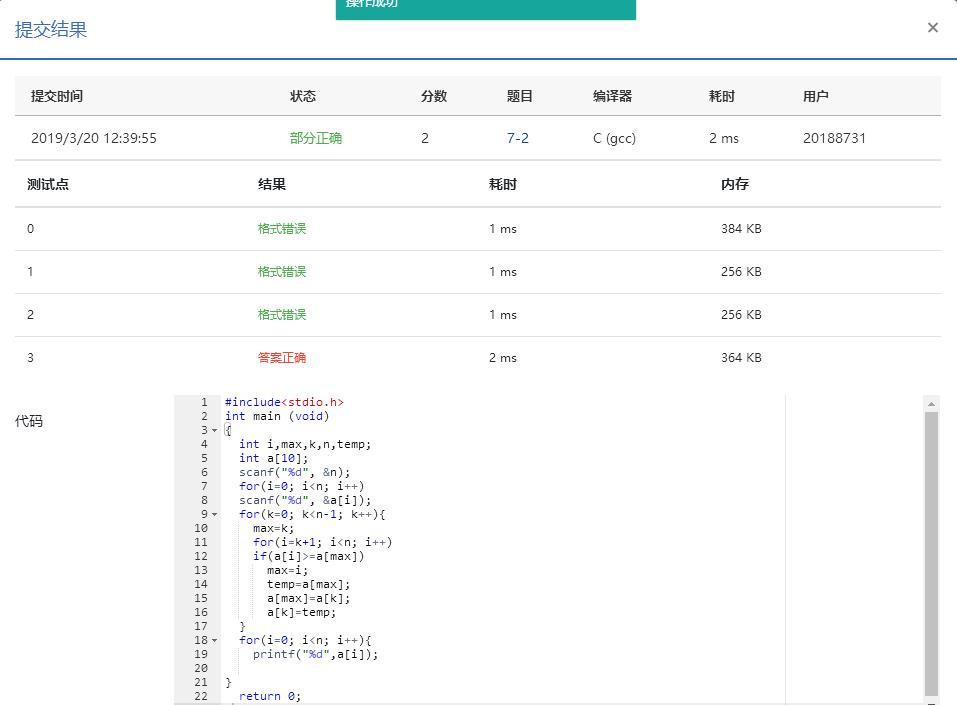## （四）运行结果截图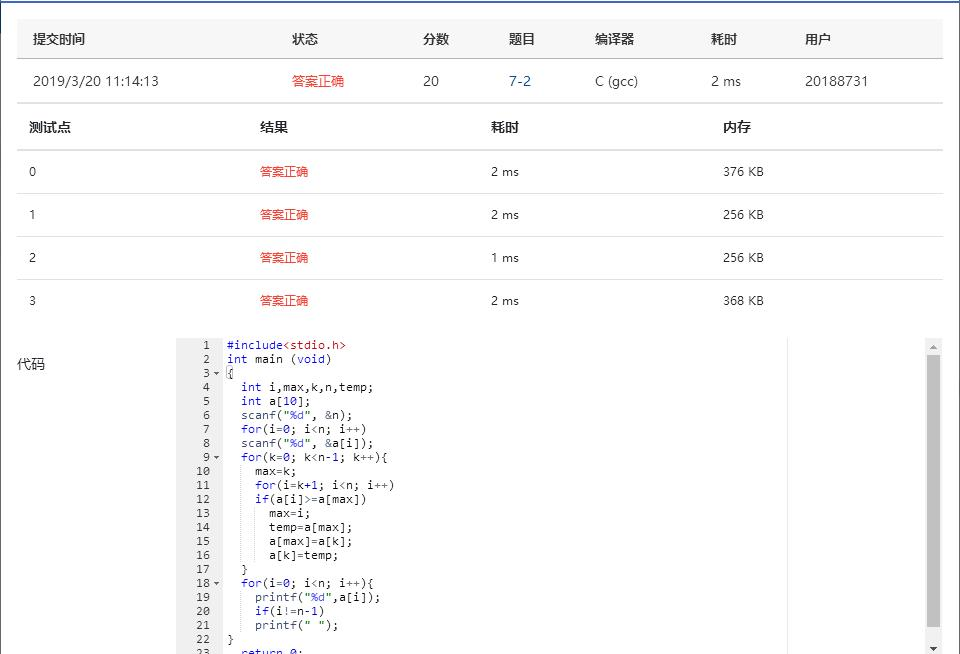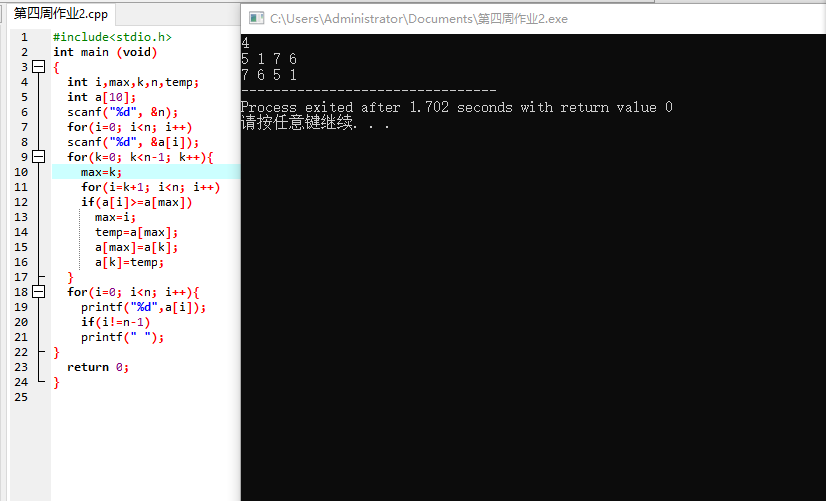# 基础作业2找鞍点

7-1 找鞍点 （20 分)

4
1 7 4 1
4 8 3 6
1 6 1 2
0 7 8 9

2 1

2
1 7
4 1

NONE

## （一）实验代码：

#include <stdio.h>
int main(void)
{
int n, i,j,min, k=0,x=0, y=0,flag=0;
int a;
scanf("%d", &n);
for(i=0; i<n; i++){
for(j=0; j<n; j++){
scanf("%d", &a[i][j]); /*遍历数组*/
}
}
for(i=0; i<n; i++){
for(j=1; j<n; j++){
if(a[x][y]<=a[i][j]){ /*给x和y赋值代替行和列*/
x=i;
y=j;
}
}
for(min=0; min<n; min++){ /*构建一个新矩阵进行比较使行最大*/
if(a[x][y]<=a[min][y]){
k++;/*满足条件用来计数*/
}
}
if(k==n){ /*满足行最大列最小*/
printf("%d %d",x,y);
flag=1;
}
k=0;
x=i+1;/*当i=0加上只有一个数的情况，也是鞍点*/
y=0;
}
if(flag!=1){
printf("NONE");
}

return 0;
}

## （二）设计思路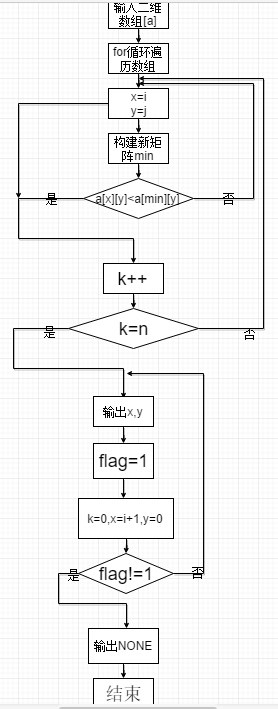## （三）实验遇到的问题及解决方案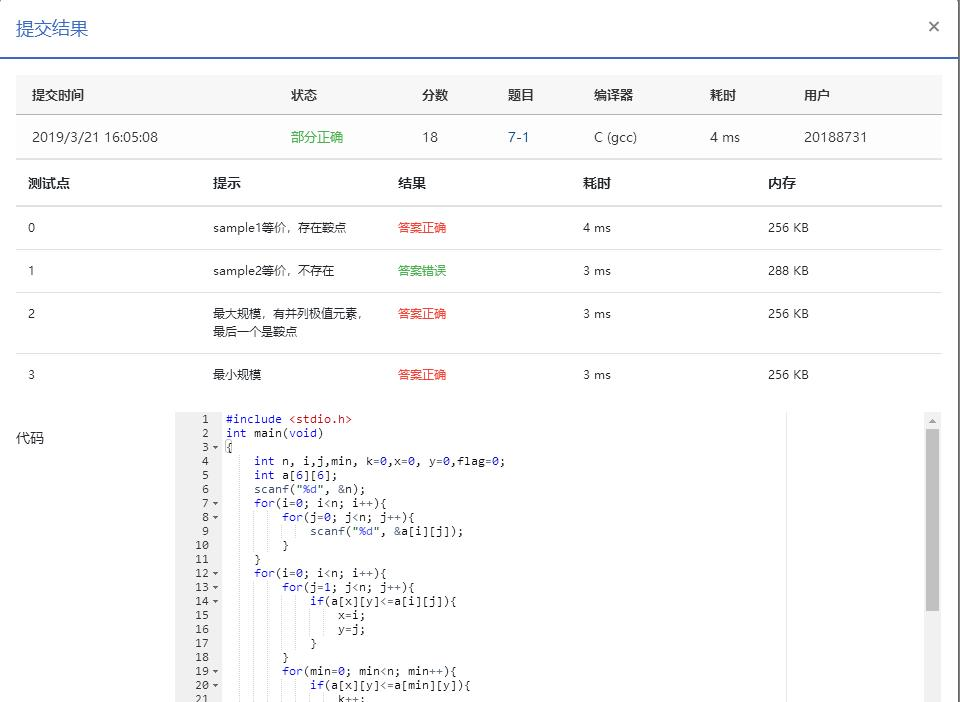## （四）运行结果截图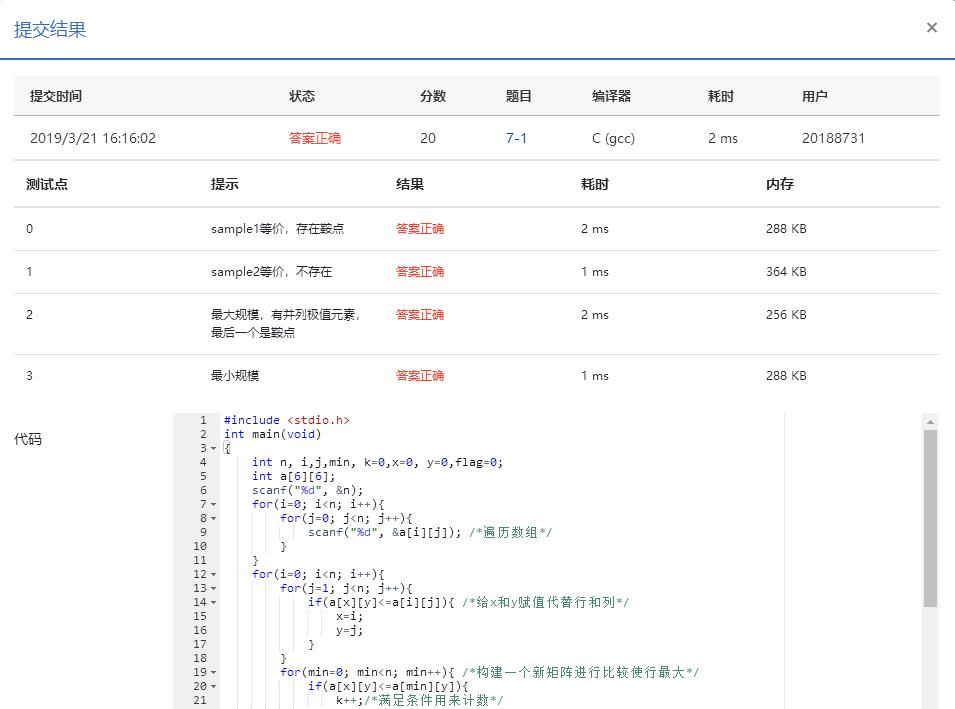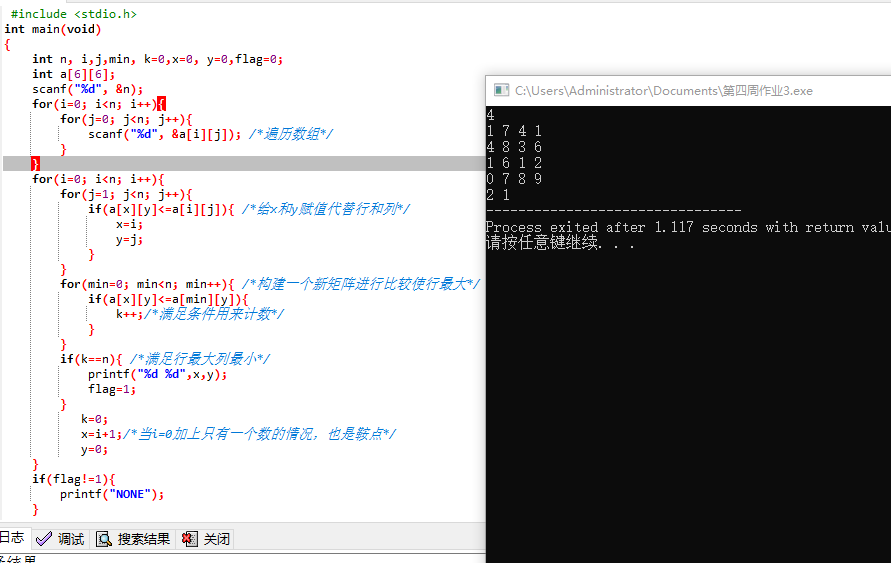# 挑战作业之冒泡排序

7-1 冒泡法排序 （10 分)

4
75 71 77 76

77 76 75 71

## （一）实验代码：

#include <stdio.h>

int main(void)
{
int n, i, j, temp;
int a;
scanf("%d", &n);

for(i = 0;i < n;i++)
{
scanf("%d", &a[i]);
}
for(i = 0;i < n;i++)
{
for(j = 0;j < n - i - 1;j++)
{
if(a[j] <= a[j+1])
{
temp = a[j];
a[j] = a[j+1];
a[j+1] = temp;
}
}
}
for(i = 0;i < n;i++)
{
printf("%d", a[i]);
if(i != n-1)
printf(" ");
}
return 0;
}

## （二）设计思路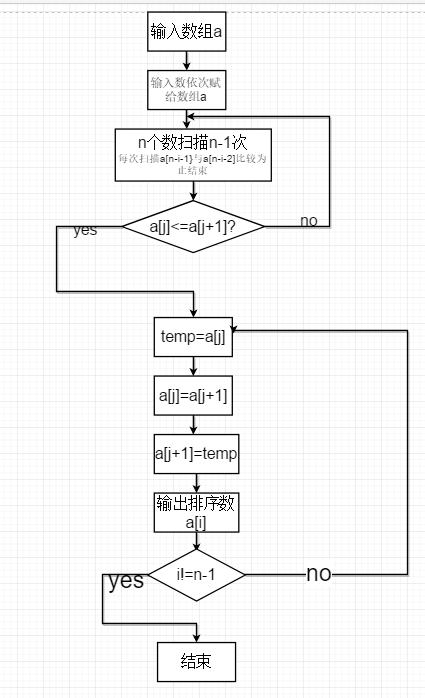## （三）实验遇到的问题及解决方案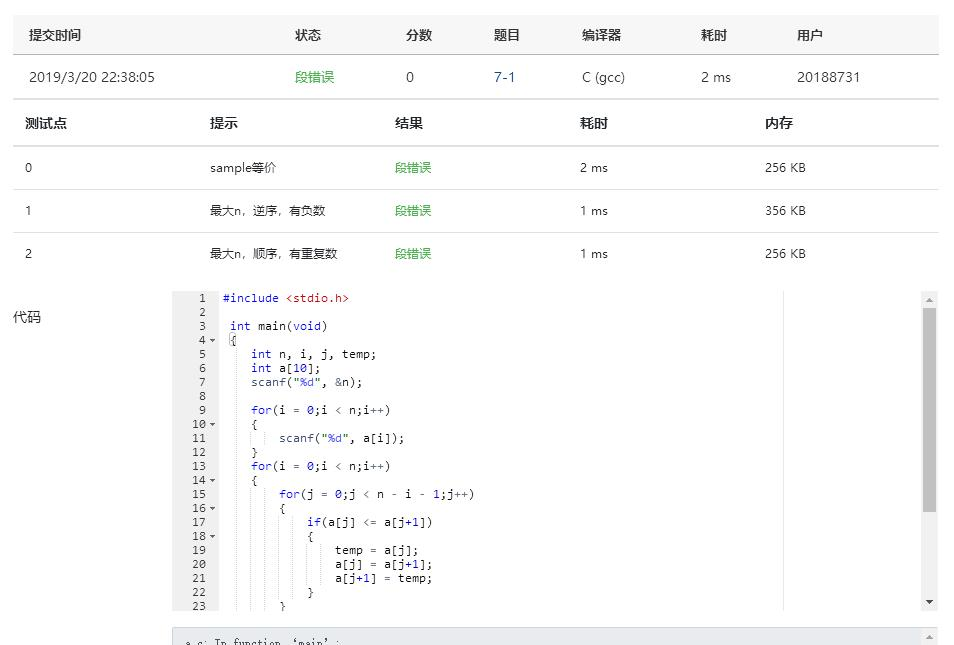## （四）运行结果截图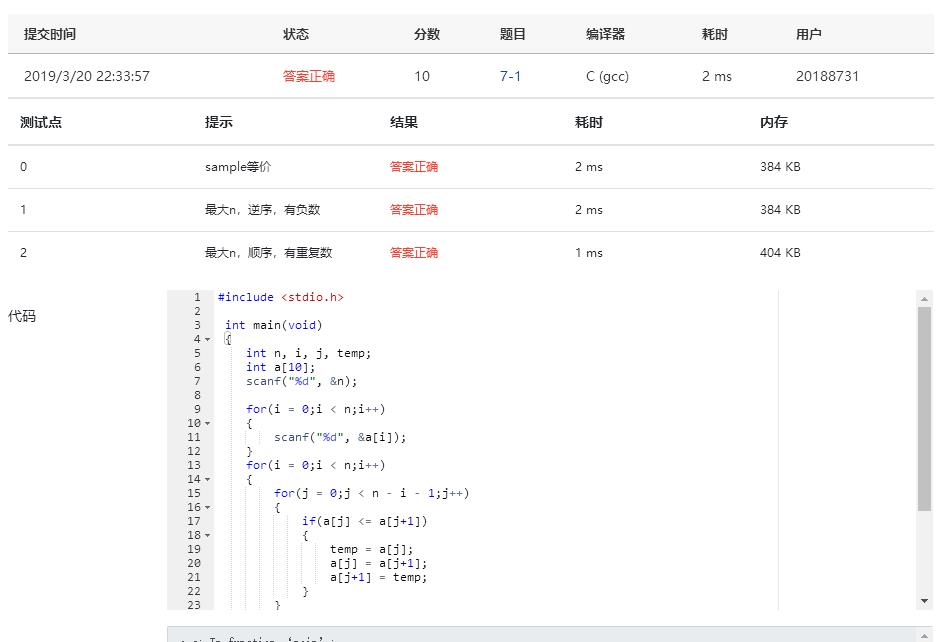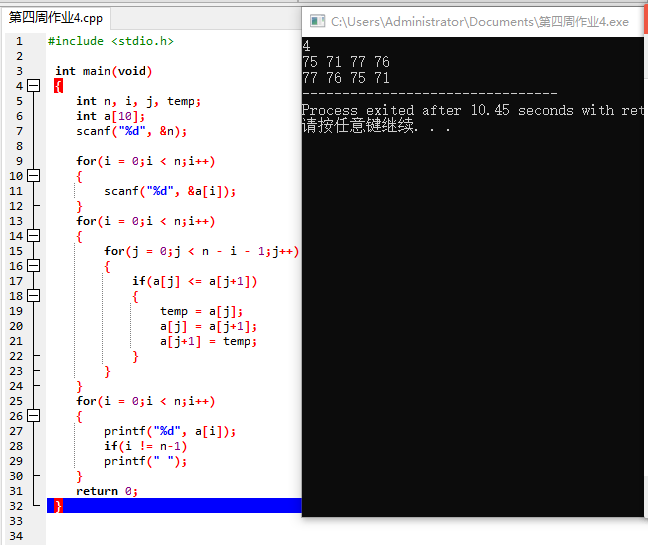### 学习进度

 周/日期 这周所花的时间 代码行 学到的知识点简介 目前比较迷惑的问题 3/17-3/21 3天 240 用一维数组进行多种方法的排序，二维数组的遍历及主副对角线的区分 找鞍点一题为什么比较数组之间的值pta通不过，找位置却可以

### 学习感悟

posted @ 2019-03-21 17:20  小嗝嗝爱作业  阅读(...)  评论(... 编辑 收藏